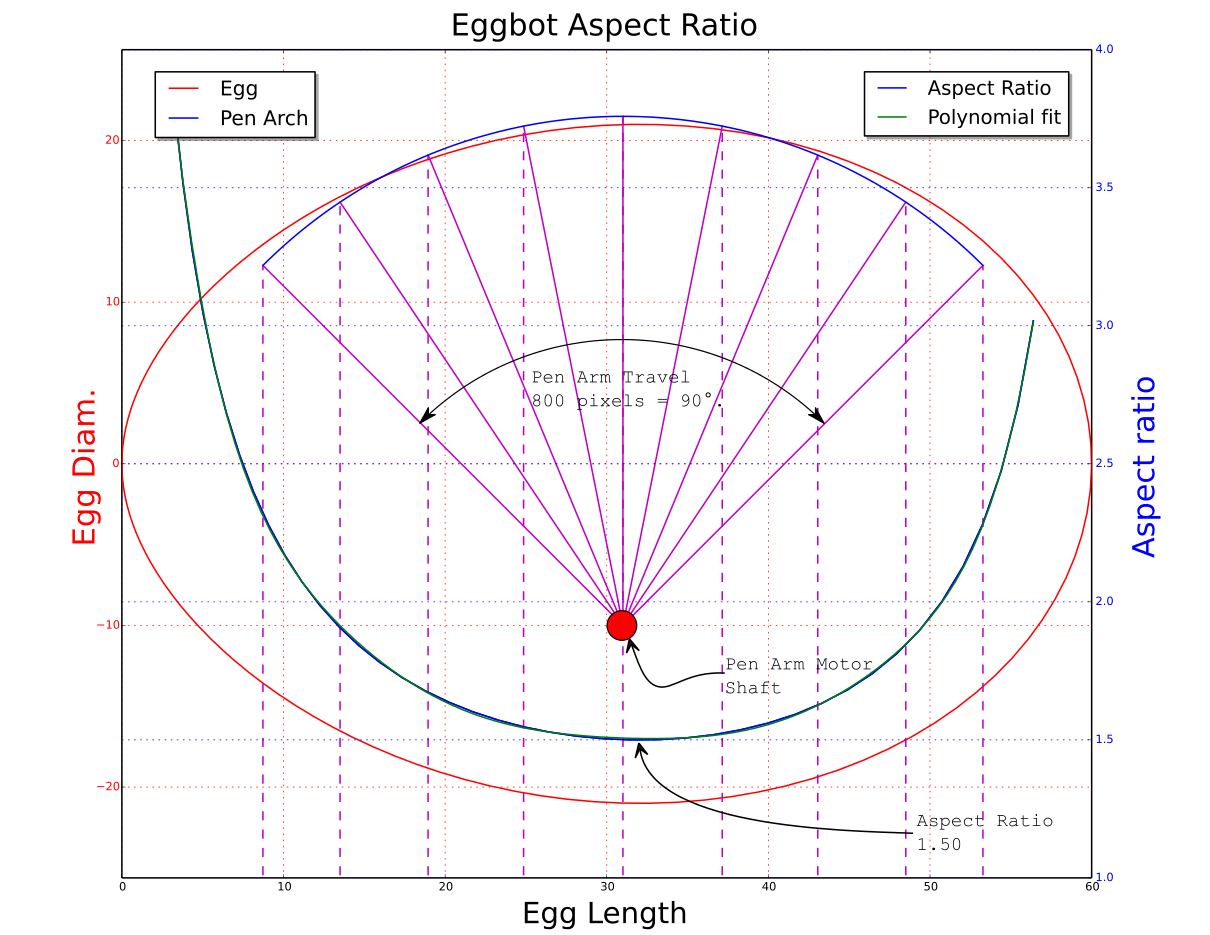# Calibration plot

Home Evil Mad Scientist Forums Egg-Bot Calibration plot

Viewing 8 posts - 1 through 8 (of 8 total)
• Author
Posts
• #20271
tsnuggs
Participant

Hi,

I’ve created what I call a ‘Calibration plot’ that I think may be helpful to others. It uses the egg bot template and creates vertical, horizontal, and diagonal lines over the entire area and also plots circles in every other square. I found it helpful to understand how plots are realized on an egg or any other shape. Specifically on an egg how things are stretched and squashed on different sections of the egg. The lines should also intersected correctly. Also, how the pen lands on the eggs makes a difference (i.e. is it perpendicular). I think it would be an excellent first plot for anyone new to the egg bot or if you think it is not plotting correctly. I would make it available on this post, but I do not see a way to attach the file.
If you are interested, I would be happy to send you the file.
Regards,
Thomas
#21505
dnewman
Participant
A good way to share the plot or plots is to post them at thingiverse.com and tag them with “eggbot”.

See also some of the plots at thingiverse.com.  E.g., the coordinate system demo plot .

#21506
ragstian
Participant

Hi.

RGDS
Ragnar

#21507
tsnuggs
Participant
Thanks for telling me about thingiverse.com.

I uploaded my file and egg photo to: http://www.thingiverse.com/thing:300570.&nbsp;

#21508
ragstian
Participant

Hi.

“404
YOU HAVE REACHED THE END OF THE THINGIVERSE.
THERE IS NOTHING AWESOME HERE…
YET.”

Nice work!

RGDS
Ragnar

#21509
ragstian
Participant

Hi.

When examining your “Calibration Plot” and really trying to understand how the diameter of the egg affects the shape of plotted objects I made a small program in python to plot the values.

I have used a term I called ‘Aspect Ratio” which is the length of a line segment made with 1° of travel along the pen axis divided by the length of a line segment made with 1° of egg rotation. The aspect ratio is plotted as a function of position on the egg.

To have a circle appear to be round in the middle of the egg it has to be drawn as an ellipsis which is 1.48 times as wide as it is tall. At the end of the eggs the value changes to around 2.4

See illustration.The plot is based on a chicken egg with 42mm diameter and 58mm length.
(To read the diameter from the plot you have to go from negative value to positive along the y-axis.) I have the pen motor shaft set 10mm lower than the egg-motor shaft and about 2mm from the middle of the egg along the length of the egg for minimum “tracking error” with this egg size.
(Red line represent the egg shape – )
XKCD Style Plot

Will make a ‘Calibration plot” based on the values from this plot for verification, stay tuned.

RGDS
Ragnar

#21510
tsnuggs
Participant

Nice graph and great info. When I created the simple calibration plot, I expected some of my circles to actually plot as circles instead of all ovals. After looking at your graph, I realize I need to adjust my pen arm motor. I’ll play around with that and see how it affects the plot. It definitely takes some experimentation to get the plots as expected.

#21511
ragstian
Participant

Hi.

As you see from the graph in the middle of the egg the “aspect ratio” is 1.5. The circles have to be ‘elongated’ 150% in order to plot as round circles on the egg. See this post as well.

Here are the text output of the plotted data;

Egg length = 58.00

At egg max diameter;
Step length pen = 0.99
Step length egg = 0.66
Aspect ratio = 1.50

L=  0.01, Inkscape y value=   0.00, aspect ratio= 6.462
L=  3.75, Inkscape y value=  50.00, aspect ratio= 3.481
L=  7.50, Inkscape y value= 100.00, aspect ratio= 2.445
L= 11.25, Inkscape y value= 150.00, aspect ratio= 2.036
L= 15.00, Inkscape y value= 200.00, aspect ratio= 1.807
L= 18.75, Inkscape y value= 250.00, aspect ratio= 1.655
L= 22.50, Inkscape y value= 300.00, aspect ratio= 1.566
L= 26.25, Inkscape y value= 350.00, aspect ratio= 1.523
L= 30.00, Inkscape y value= 400.00, aspect ratio= 1.507
L= 33.75, Inkscape y value= 450.00, aspect ratio= 1.508
L= 37.50, Inkscape y value= 500.00, aspect ratio= 1.538
L= 41.25, Inkscape y value= 550.00, aspect ratio= 1.615
L= 45.00, Inkscape y value= 600.00, aspect ratio= 1.751
L= 48.75, Inkscape y value= 650.00, aspect ratio= 1.974
L= 52.50, Inkscape y value= 700.00, aspect ratio= 2.458
L= 56.25, Inkscape y value= 750.00, aspect ratio= 3.843

RGDS
Ragnar

Viewing 8 posts - 1 through 8 (of 8 total)
• You must be logged in to reply to this topic.